﻿ 行星轮偏心误差对行星齿轮传动系统均载性能的影响 Study on the Influence Rules of Planetary Gear Eccentric Errors on Dynamic Load Sharing Behavior of a Planetary Gear Train

Mechanical Engineering and Technology
Vol.07 No.02(2018), Article ID:24481,7 pages
10.12677/MET.2018.72013

Study on the Influence Rules of Planetary Gear Eccentric Errors on Dynamic Load Sharing Behavior of a Planetary Gear Train

Shangzhen Sun1, Aimin Yao1, Tongjie Li2*, Jie Ma2, Juan Wang2

1Bengbu Planet Engineering Machinery Co. Ltd, Bengbu Anhui

2College of Mechanical Engineering, Anhui Science & Technology University, Chuzhou Anhui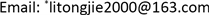Received: Mar. 27th, 2018; accepted: Apr. 17th, 2018; published: Apr. 24th, 2018ABSTRACT

By using the method of numerical simulation, the influence rules of planetary gear eccentric errors on dynamic load sharing behavior of a planetary gear train are studied based on a nonlinear lateral-torsional vibration model. Under the assumption that all of the planetary gears have the same eccentric errors, planetary gear train has a threshold value error; the dynamic load sharing behavior of the system will remain unchanged with the eccentric error of planetary gears changing under the threshold value error; the dynamic load sharing behavior of the system will worsen significantly with the eccentric error of planetary gears increase above the threshold value error.

Keywords:Planetary Gear Train, Nonlinear Lateral-Torsional Vibration Model, Planetary Gear Eccentric Errors, Dynamic Load Sharing Behavior

1蚌埠市行星工程机械有限公司，安徽 蚌埠

2安徽科技学院机械工程学院，安徽 滁州1. 引言

2. 动力学模型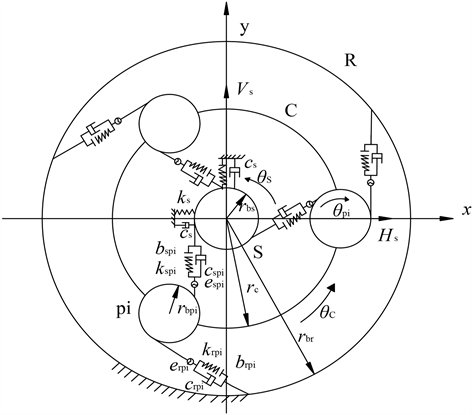Figure 1. Bending-torsional coupling nonlinear dynamic model of planetary gear train

$\left\{\begin{array}{l}{e}_{\text{sp}i}\left(t\right)={A}_{\text{sp}i}\mathrm{sin}\left(\omega t+{\beta }_{\text{s}i}\right)+{E}_{\text{s}}\mathrm{sin}\left({\omega }_{\text{sc}}t+{\beta }_{\text{s}}-\frac{2\text{π}\left(i-1\right)}{N}+\alpha \right)+{E}_{\text{p}i}\mathrm{sin}\left({\omega }_{\text{pc}}t+{\beta }_{\text{p}i}+\alpha \right)\\ {e}_{\text{rp}i}\left(t\right)={A}_{\text{rp}i}\mathrm{sin}\left(\omega t+{\beta }_{\text{r}i}\right)+{E}_{\text{p}i}\mathrm{sin}\left({\omega }_{\text{pc}}t+{\beta }_{\text{p}i}+\alpha \right)-{E}_{\text{r}}\mathrm{sin}\left({\omega }_{\text{rc}}t+{\beta }_{\text{r}}-\frac{2\text{π}\left(i-1\right)}{N}+\alpha \right)\end{array}$ , $i=1,2,\cdots ,N$ (1)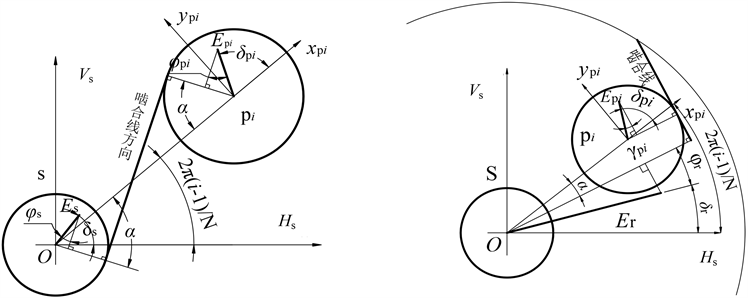(a) 偏心误差与外啮合线的投影关系 (b) 偏心误差与内啮合线的投影关系

Figure 2. Geometrical relationship of eccentricity error in gear pair(2)

 (3)

$\left\{\begin{array}{l}{F}_{\text{sp}i}^{p}={k}_{\text{sp}i}\left(t\right)f\left({X}_{\text{sp}i},{b}_{\text{sp}i}\right)\\ {F}_{\text{rp}i}^{p}={k}_{\text{rp}i}\left(t\right)f\left({X}_{\text{rp}i},{b}_{\text{rp}i}\right)\end{array}$ (4)

3. 均载性能的考评

t时刻系统的第i个行星齿轮分配的载荷与该时刻N个行星轮的平均载荷的比值即为该时刻该行星轮的动态均载系数，其外内啮合副各行星轮的动态均载系数分别以 ${\Omega }_{\text{sp}i}\left(t\right)$${\Omega }_{\text{rp}i}\left(t\right)$ 表示

$\left\{\begin{array}{l}{\Omega }_{\text{spi}}\left(t\right)=N×{F}_{\text{sp}i}^{p}\left(t\right)/\sum _{i=1}^{N}{F}_{\text{sp}i}^{p}\left(t\right)\\ {\Omega }_{\text{rp}i}\left(t\right)=N×{F}_{\text{rp}i}^{p}\left(t\right)/\sum _{i=1}^{N}{F}_{\text{rp}i}^{p}\left(t\right)\end{array}$ (5)

$\left\{\begin{array}{l}{\Omega }_{\text{s}}\left(t\right)=N×\mathrm{max}\left({\Omega }_{\text{sp}i}\left(t\right)\right)/\sum _{i=1}^{N}{\Omega }_{\text{sp}i}\left(t\right)\\ {\Omega }_{\text{r}}\left(t\right)=N×\mathrm{max}\left({\Omega }_{\text{rp}i}\left(t\right)\right)/\sum _{i=1}^{N}{\Omega }_{\text{sp}i}\left(t\right)\end{array}$ (6)

$\left\{\begin{array}{l}{\Omega }_{\text{s}}=\mathrm{max}\left({\Omega }_{\text{s}}\left(t\right)\right)\\ {\Omega }_{\text{r}}=\mathrm{max}\left({\Omega }_{\text{r}}\left(t\right)\right)\end{array},\text{\hspace{0.17em}}t\in \left[0~T\right]$ (7)

4. 行星轮偏心误对均载性能的影响规律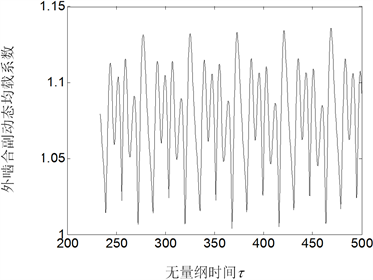Figure 3. Load sharing coefficient of every external gear pair when Epi = 1 × 106 m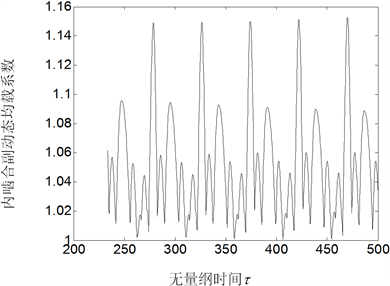Figure 4. Load sharing coefficient of every internal gear pair when Epi = 1 × 106 m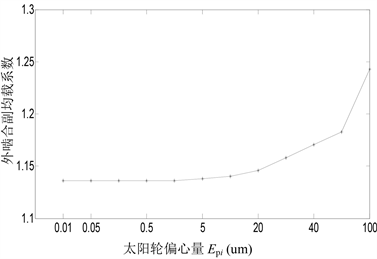Figure 5. Load sharing coefficient of external gear pair with the eccentricity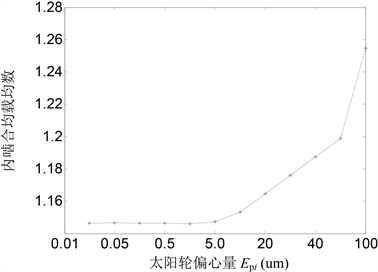Figure 6. Load sharing coefficient of internal gear pair with the eccentricity

5. 结论

1) 存在一个行星轮偏心误差阀值，当Epi小于该阀值时，Epi的变化对行星齿轮传动系统的均载性能没有明显的影响；当Epi大于该阀值时，Epi的增大会显著削弱行星齿轮传动系统的均载性能。

2) 机械设计时，要注意通过控制齿轮精度的手段使行星轮偏心误差处于该误差阀值之下以保证行星齿轮传动系统的均载性能。误差阀值可以通过大量的数值试验获得。

Study on the Influence Rules of Planetary Gear Eccentric Errors on Dynamic Load Sharing Behavior of a Planetary Gear Train[J]. 机械工程与技术, 2018, 07(02): 105-111. https://doi.org/10.12677/MET.2018.72013

1. 1. 叶福民, 朱如鹏, 鲍和云, 等. 非等模数非等压力角行星齿轮系的动力学均载特性[J]. 华南理工大学学报, 2011, 39(1): 117-123.

2. 2. 方宗德, 沈允文, 黄镇东. 2K-H行星减速器的动态特性[J]. 西北工业大学学报, 1990, 10(4): 361-371.

3. 3. 陆俊华, 朱如鹏, 靳广虎. 行星传动动态均载特性分析[J]. 机械工程学报, 2009, 45(5): 85-90.

4. 4. 朱增宝, 朱如鹏, 李应生, 等. 安装误差对封闭差动人字齿轮传动系统动态均载特性的影响[J]. 机械工程学报, 2012, 48(3): 16-24.

5. 5. 周建星, 董海军. 基于非线性动力学的行星传动均载性能研究[J]. 机械科学与技术, 2008, 27(6): 808-811

6. 6. 李同杰, 朱如鹏, 鲍和云, 等. 行星齿轮系扭转非线性振动建模与运动分岔特性研究[J]. 机械工程学报, 2011, 47(21): 76-83.